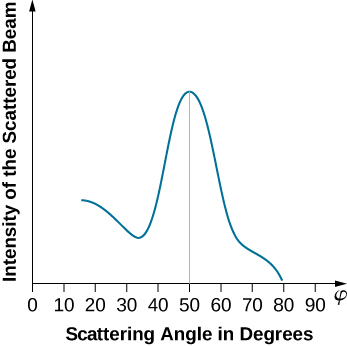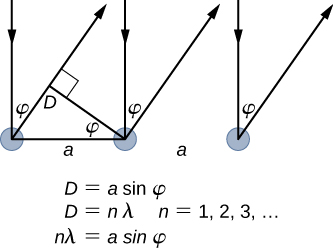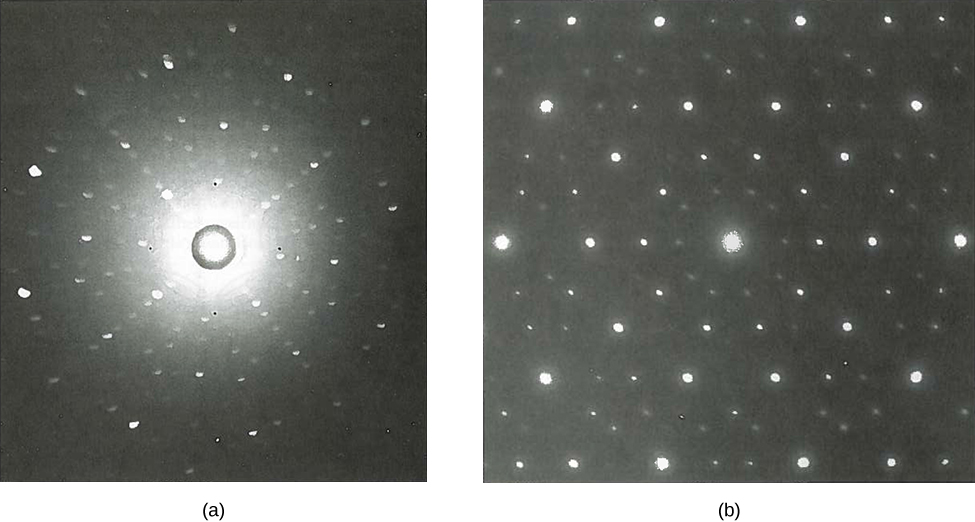# 6.5 De broglie’s matter waves  (Page 4/10)

 Page 4 / 10

The lattice spacing of the Davisson–Germer target, determined with X-ray crystallography, was measured to be $a=2.15\phantom{\rule{0.2em}{0ex}}\text{Å}.$ Unlike X-ray crystallography in which X-rays penetrate the sample, in the original Davisson–Germer experiment, only the surface atoms interact with the incident electron beam. For the surface diffraction, the maximum intensity of the reflected electron beam is observed for scattering angles that satisfy the condition $n\lambda =a\text{sin}\phi$ (see [link] ). The first-order maximum (for $n=1$ ) is measured at a scattering angle of $\phi \approx 50\text{°}$ at $\text{Δ}V\approx 54\text{V},$ which gives the wavelength of the incident radiation as $\lambda =\left(2.15\phantom{\rule{0.2em}{0ex}}\text{Å}\right)\text{sin}50\text{°}=1.64\phantom{\rule{0.2em}{0ex}}\text{Å}.$ On the other hand, a 54-V potential accelerates the incident electrons to kinetic energies of $K=54\phantom{\rule{0.2em}{0ex}}\text{eV}.$ Their momentum, calculated from [link] , is $p=2.478\phantom{\rule{0.2em}{0ex}}×\phantom{\rule{0.2em}{0ex}}{10}^{-5}\text{eV}·\text{s}\phantom{\rule{0.1em}{0ex}}\text{/}\phantom{\rule{0.1em}{0ex}}\text{m}.$ When we substitute this result in [link] , the de Broglie wavelength is obtained as

$\lambda =\frac{h}{p}=\frac{4.136\phantom{\rule{0.2em}{0ex}}×\phantom{\rule{0.2em}{0ex}}{10}^{-15}\text{eV}·\text{s}}{2.478\phantom{\rule{0.2em}{0ex}}×\phantom{\rule{0.2em}{0ex}}{10}^{-5}\text{eV}·\text{s}\phantom{\rule{0.1em}{0ex}}\text{/}\phantom{\rule{0.1em}{0ex}}\text{m}}=1.67\phantom{\rule{0.2em}{0ex}}\text{Å}.$

The same result is obtained when we use $K=54\phantom{\rule{0.2em}{0ex}}\text{eV}$ in [link] . The proximity of this theoretical result to the Davisson–Germer experimental value of $\lambda =1.64\phantom{\rule{0.2em}{0ex}}\text{Å}$ is a convincing argument for the existence of de Broglie matter waves.The experimental results of electron diffraction on a nickel target for the accelerating potential in the electron gun of about Δ V = 54 V : The intensity maximum is registered at the scattering angle of about φ = 50 ° .In the surface diffraction of a monochromatic electromagnetic wave on a crystalline lattice structure, the in-phase incident beams are reflected from atoms on the surface. A ray reflected from the left atom travels an additional distance D = a sin φ to the detector, where a is the lattice spacing. The reflected beams remain in-phase when D is an integer multiple of their wavelength λ . The intensity of the reflected waves has pronounced maxima for angles φ satisfying n λ = a sin φ .

Diffraction lines measured with low-energy electrons, such as those used in the Davisson–Germer experiment, are quite broad (see [link] ) because the incident electrons are scattered only from the surface. The resolution of diffraction images greatly improves when a higher-energy electron beam passes through a thin metal foil. This occurs because the diffraction image is created by scattering off many crystalline planes inside the volume, and the maxima produced in scattering at Bragg angles are sharp (see [link] ).Diffraction patterns obtained in scattering on a crystalline solid: (a) with X-rays, and (b) with electrons. The observed pattern reflects the symmetry of the crystalline structure of the sample.

Since the work of Davisson and Germer, de Broglie’s hypothesis has been extensively tested with various experimental techniques, and the existence of de Broglie waves has been confirmed for numerous elementary particles. Neutrons have been used in scattering experiments to determine crystalline structures of solids from interference patterns formed by neutron matter waves. The neutron has zero charge and its mass is comparable with the mass of a positively charged proton. Both neutrons and protons can be seen as matter waves. Therefore, the property of being a matter wave is not specific to electrically charged particles but is true of all particles in motion. Matter waves of molecules as large as carbon ${C}_{60}$ have been measured. All physical objects, small or large, have an associated matter wave as long as they remain in motion. The universal character of de Broglie matter waves is firmly established.

If you lie on a beach looking at the water with your head tipped slightly sideways, your polarized sunglasses do not work very well.Why not?
it has everything to do with the angle the UV sunlight strikes your sunglasses.
Jallal
this is known as optical physics. it describes how visible light, ultraviolet light and infrared light interact when they come into contact with physical matter. usually the photons or light upon interaction result in either reflection refraction diffraction or interference of the light.
Jallal
I hope I'm clear if I'm not please tell me to clarify further or rephrase
Jallal
what is bohrs model for hydrogen atom
hi
Tr
Hello
Youte
Hi
Nwangwu-ike
hi
Siddiquee
hi
Omar
helo
Mcjoi
what is the value of speed of light
1.79×10_¹⁹ km per hour
Swagatika
what r dwarf planet
what is energy
কাজের একক কী
Jasim
কাজের একক কী
Jasim
friction ka direction Kaise pata karte hai
friction is always in the opposite of the direction of moving object
Punia
A twin paradox in the special theory of relativity arises due to.....? a) asymmetric of time only b) symmetric of time only c) only time
b) symmetric of time only
Swagatika
fundamental note of a vibrating string
every matter made up of particles and particles are also subdivided which are themselves subdivided and so on ,and the basic and smallest smallest smallest division is energy which vibrates to become particles and thats why particles have wave nature
Alvin
what are matter waves? Give some examples
according to de Broglie any matter particles by attaining the higher velocity as compared to light'ill show the wave nature and equation of wave will applicable on it but in practical life people see it is impossible however it is practicaly true and possible while looking at the earth matter at far
Manikant
a centeral part of theory of quantum mechanics example:just like a beam of light or a water wave
Swagatika
Mathematical expression of principle of relativity
given that the velocity v of wave depends on the tension f in the spring, it's length 'I' and it's mass 'm'. derive using dimension the equation of the wave
What is the importance of de-broglie's wavelength?
he related wave to matter
Zahid
at subatomic level wave and matter are associated. this refering to mass energy equivalence
Zahid
it is key of quantum
Manikant
how those weight effect a stable motion at equilibrium
how do I differentiate this equation- A sinwt with respect to t
just use the chain rule : let u =wt , the dy/dt = dy/du × du/dt : wA × cos(wt)
Jerry
I see my message got garbled , anyway use the chain rule with u= wt , etc...
Jerry
de broglie wave equation
vy beautiful equation
chandrasekharByBy Donyea SweetsBy Michael SagBy Dan ArielyBy Tod McGrathBy OpenStaxBy Madison ChristianBy Miranda ReisingBy Michael SagBy Mahee Boo